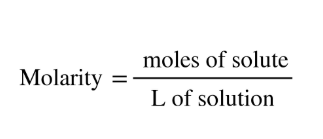# Problem: A chemist prepares a solution of aluminum chloride (AICI 3) by measuring out 24.6 g of aluminum chloride into a 250. mL volumetric flask and filling the flask to the mark with water. Calculate the concentration in mol/L of the chemist's aluminum chloride solution. Be sure your answer has the correct number of significant digits.

###### FREE Expert Solution
100% (313 ratings)
###### FREE Expert Solution

Molarity can be calculated as:For this problem, we are given the mass of AlCl3, we can calculate the moles by using its molar mass which is:

MM of AlCl3:

Al - 26.98 (1) = 26.98

Cl - 35.45 (3) = 106.36

26.98 + 106.35 = 133.34 g/mol

100% (313 ratings)###### Problem Details

A chemist prepares a solution of aluminum chloride (AICI 3) by measuring out 24.6 g of aluminum chloride into a 250. mL volumetric flask and filling the flask to the mark with water. Calculate the concentration in mol/L of the chemist's aluminum chloride solution. Be sure your answer has the correct number of significant digits.

What scientific concept do you need to know in order to solve this problem?

Our tutors have indicated that to solve this problem you will need to apply the Molarity concept. You can view video lessons to learn Molarity. Or if you need more Molarity practice, you can also practice Molarity practice problems.

What is the difficulty of this problem?

Our tutors rated the difficulty ofA chemist prepares a solution of aluminum chloride (AICI 3) ...as medium difficulty.

How long does this problem take to solve?

Our expert Chemistry tutor, Dasha took 4 minutes and 34 seconds to solve this problem. You can follow their steps in the video explanation above.

What professor is this problem relevant for?

Based on our data, we think this problem is relevant for Professor Hopkins' class at LSU.